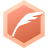# 女友叫我证明此题，难倒我了！大可山人博客专家认证
2003-07-18 04:42:50
0<= C <=1,
0<= K <=1,

...全文
26 20 打赏 收藏 举报

20 条回复

djzblue 2003-07-18
• 打赏
• 举报

loulanlouzhu 2003-07-18
• 打赏
• 举报

xiaomimamaya 2003-07-18
• 打赏
• 举报

loulanlouzhu 2003-07-18
• 打赏
• 举报

Y＝C*(1-k)+K

y＝c*(1-c)+c=-(c-1)2+1-->注：此处为c-1的平方

0=<c-1的平方=<1;
-1=<z<=0;
0=<z+1<=1;

• 打赏
• 举报

C（青色）M（洋红）Y（黄）K（黑色）
R=1-Min(1,C*(1-K)+K);
G=1-Min(1,M*(1-K)+K);
B=1-Min(1,Y*(1-K)+K);

R*255=RED（红色）
G*255=GREEN（绿色）
B*255=BLUE（蓝色）

R=(1-K)*(1-C);

0<=C*(1-K)+K<=1
2mao 2003-07-18
• 打赏
• 举报

EternalBlue 2003-07-18
• 打赏
• 举报

2mao 2003-07-18
• 打赏
• 举报

=1-(1-k)+c*(1-k)
=1-(1-c)(1-k) ----------*
becase
0<=1-c<=1
0<=1-k<=1
then
0<=(1-c)(1-k)<=1
so 0<=*<=1

• 打赏
• 举报

lczddd(李找乐):

ccchixin 2003-07-18
• 打赏
• 举报

0<=c<=1
c*(1-k)<=1*(1-k)
c*(1-K)+k<=1

0<=c<=1
0<=ck<=k
ck<=k+c
c*(1-k)+k>=0

lczddd 2003-07-18
• 打赏
• 举报

0<= C <=1

0<= K <=1

0<= 1-k <=1

0<= c*(1-k) <=1

must be it!!!
lczddd 2003-07-18
• 打赏
• 举报

0<= C <=1

0<= K <=1

0<1-k<1

0<c*(1-k)<1

cxq1846 2003-07-18
• 打赏
• 举报

1 >= c >= 0 并且1 >= (1-k) >= 0(已证）=> (1-k) * 1 >= c * (1-k) =>(1-k) >=c *(1-k)
=> 1 >= c * (1-k) + k

panyee 2003-07-18
• 打赏
• 举报

c = 1 ,
k = 2,
1*(1-0)+1 = 2;

c=0,
k=0,
0*(1-0)+0=0

c=1,k=0
1*(1-0)+0=1;

c=0,k=1
0*(1-1)+1=1

ccchixin 2003-07-18
• 打赏
• 举报

ETstudio 2003-07-18
• 打赏
• 举报

C-C*k+k
=C+K-C*K

0<=C*K<=1高中历史之历史百科北宋皇帝亲自出二道科举题难倒无数“高考”才子素材c语言编程vingt,讲真，学法语这么久，这道题难倒我了……笔试面试的小结 反leetcode001：3sum solution
leetcode网站，提供了一些算法题，还有内嵌了c++和java的编辑器，提供运行测试。 所以决定弥补自己算法的不足，多学习多思考实现。 第一道题就难倒我了= =||题目如下 3Sum Given an array S of n integers, are there elements a, b, c in S such that a + b + c = 0?algorithm chapter8 8.15